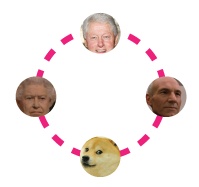# Stable particle ring - JEEFour particles, each of mass $M$ are equidistant from each other in 3 dimensional space. They each move along a circle of radius R, under the action of their mutual gravitational attraction. The speed of each particle is:

$\begin{array} { l l l } & (1) \frac{1}{2} \sqrt{ \frac{GM}{R} ( 1 + 2 \sqrt{2}) } \qquad \qquad & (2) \sqrt{ \frac{ GM } {R} } \\ & (3) \sqrt{ 2 \sqrt{2} \frac{ GM}{R} } & (4) \sqrt{ \frac{GM}{R} ( 1 + 2 \sqrt{2} ) } \\ \end{array}$

Choose the number which corresponds to the correct answer.

×

Problem Loading...

Note Loading...

Set Loading...